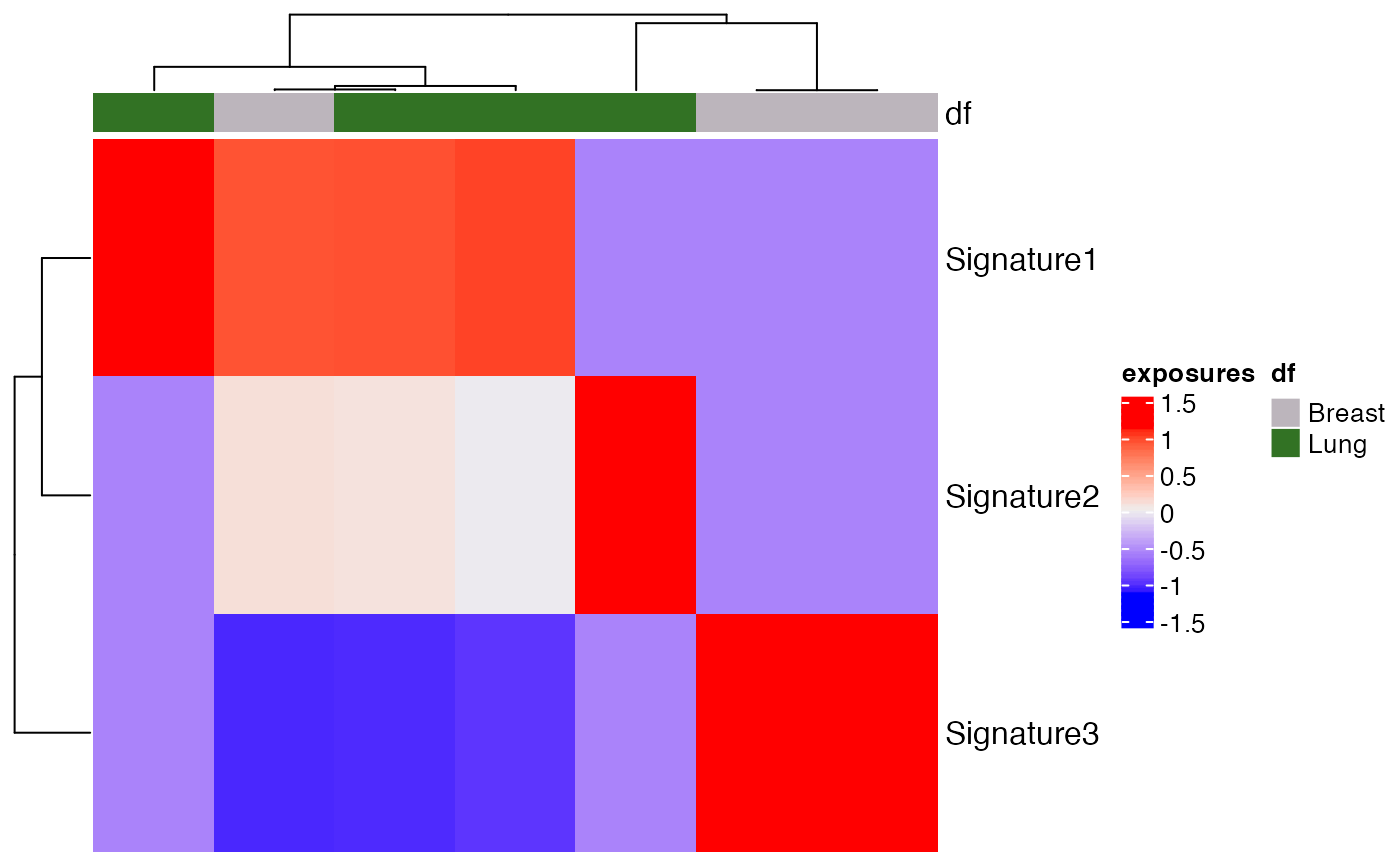The exposures for different signatures can be visualized using a heatmap with this function. Heatmaps make it easier to visualize the data by representing the magnitude of exposure values as color in 2-dimensions. The variation in color intensity can help see if the exposures are clustered or how they vary over space. Exposures can be normalized by providing the proportional argument. Column annotations can also be seen by passing the col_annot argument.

plot_heatmap(
res_annot,
proportional = FALSE,
show_column_names = FALSE,
show_row_names = TRUE,
scale = TRUE,
subset_tumor = NULL,
subset_signatures = NULL,
annotation = NULL,
...
)

Arguments

res_annot A musica_result object generated by a mutational discovery or prediction tool. If TRUE, then the exposures will be normalized to between 0 and 1 by dividing by the total number of counts for each sample. Default FALSE. Boolean check. If True, column names are shown. Otherwise, they aren't. Default FALSE Boolean check. If True, row names are shown. Otherwise, they aren't. Default FALSE Boolean check. If True, values are scaled by z-score. Otherwise, they aren't. Default TRUE Users can specify certain tumor types on which they want to subset the exposure matrix for plotting the heatmap. Users can specify certain signatures on which they want to subset the exposure matrix plotting the heatmap. Users have the option of plotting the exposure matrix based on their given annotation like Tumor_Subtypes or age. Error given if the user given annotation doesn't exist in the res_annot annotation object. Ellipsis used for passing any arguments directly to the ComplexHeatmap's heatmap function.

Value

Generates a heatmap for using the exposure matrix.

Examples

data(res_annot) plot_heatmap(res_annot = res_annot, proportional = TRUE, scale = TRUE, annotation = "Tumor_Subtypes")